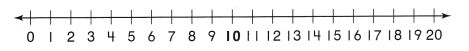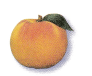Refer to our Texas Go Math Grade 2 Answer Key Pdf to score good marks in the exams. Test yourself by practicing the problems from Texas Go Math Grade 2 Module 5 Assessment Answer Key.

Vocabulary

Question 1.
Circle the sum. (p.148)
3 + 8 = 11

Question 2.
Circle the difference. (p.160)
14 – 9 = 5

Concepts arid Skills

Make a ten to find the sum. Write the sum. (TEKS 2.4.A)

Question 3.
9 + 7 __________
10 + ________ = _________

Question 4.
6 + 8 = ___________
10 + ___________ = ___________

Show the tens fact you used. Write the difference. (TEKS 2.4.A)Question 5.
12 – 6 = ___________
10 – ___________ = ___________

Question 6.
16 – 7 = ___________
10 – ___________ = ___________

Write the sum or difference. (TEKS 2.4.A)

Question 7.
8 + 4 = __________

Question 8.
________ = 6 + 5

Question 9.
9 + 1 = ___________

Question 10.
11 – 3 = ___________

Question 11.
_______ = 9 – 7

Question 12.
13 – 8 = ___________

Fill in the bubble for the correct answer choice.

Question 13.
Rachel put 5 peaches in a basket. Her mom put twice as many peaches in the basket. How many peaches are in the basket now? (TEKS 2.4.A)(A) 15
(B) 5
(C) 10

Question 14.
Hudson had 13 grapes. He gave 5 grapes to his sister and 3 grapes to his brother. How many grapes does Hudson have now? (TEKS 2.4.A)
(A) 18
(B) 5
(C) 8

Question 15.
There were 9 apples in a bag. Erin took some apples out of the bag. Now there are 6 apples in the bag. Which number sentence can be used to find the number of apples that Erin took out of the bag? (TEKS 2.4.A, 2.4.C)
(A) 9 – ☐ = 6
(B) 9 + 6 = ☐
(C) ☐ + 2 = 6

Question 16.
Tina has some blue markers and 6 green markers. She has 12 markers altogether. How many blue markers does Tina have? (TEKS 2.4.A)(A) 8
(B) 18
(C) 6